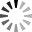# 3. Visual editor and strategy designer blocks - 2

3. Visual editor and strategy designer blocks - 2VideoUnavailable
The lesson covers:
• Description of the cube Variable and examines examples of the use of the cube
• Examines the Logic cube and an example of its application
• Examines the Previous value cube and an example of its application
• Examines the Mathematics section and the use of cubes that are included in it
• Examines the Converters section (cubes "Indexer" and "Converter") and an example of sharing the cubes of the section
• Considers the cube "Opening a position"
• Considers the cube "Position" and the scheme in which it is applied
• Considers the cube "Comparison" and an example of its application
• Considered building a simple strategy:
If the closing price of the previous candle is less than the closing price of the current one, then we buy, if not, we sell.
• Considers the "Comparison" cube and an example of its application

(In the fifth version, the mathematics section is replaced by one formula cube. In the fifth version of the program, the "Position opening" cube is replaced with "Position registration")

Designer schemas for this lesson

 Thanks: Benoit Serratrice

Attach files by dragging & dropping, , or pasting from the clipboard.